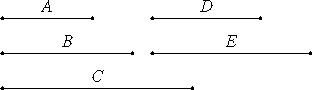# Proposition 32

To find two medial straight lines commensurable in square only, containing a medial rectangle, such that the square on the greater is greater than the square on the less by the square on a straight line commensurable with the greater.
X.29

Set out three rational straight lines A, B, and C commensurable in square only, such that the square on A is greater than the square on C by the square on a straight line commensurable with A. Let the square on D equal the rectangle A by B.

X.21

Then the square on D is medial. Therefore D is also medial.

Let the rectangle D by E equal the rectangle B by C.

Then since as the rectangle A by is is to the rectangle B by C as A is to C, while the square on D equals the rectangle A by B, and the rectangle D by E equals the rectangle B by C, therefore A is to C as the square on D is to the rectangle D by E.X.11

But the square on D is to the rectangle D by E as D is to E, therefore A is to C as D is to E. But A is commensurable with C in square only, therefore D is also commensurable with E in square only.

X.23,Note

But D is medial, therefore E is also medial.

X.14

And, since A is to C as D is to E, while the square on A is greater than the square on C by the square on a straight line commensurable with A, therefore the square on D is greater than the square on E by the square on a straight line commensurable with D.

I say next that the rectangle D by E is also medial.

X.21

Since the rectangle B by C equals the rectangle D by E, while the rectangle B by C is medial, therefore the rectangle D by E is also medial.

Therefore two medial straight lines D and E, commensurable in square only, and containing a medial rectangle, have been found such that the square on the greater is greater than the square on the less by the square on a straight line commensurable with the greater.

X.30

Similarly again it can be proved that the square on D is greater than the square on E by the square on a straight line incommensurable with D when the square on A is greater than the square on C by the square on a straight line incommensurable with A.

Q.E.D.

## Guide

This proposition is not used in the rest of the Elements.